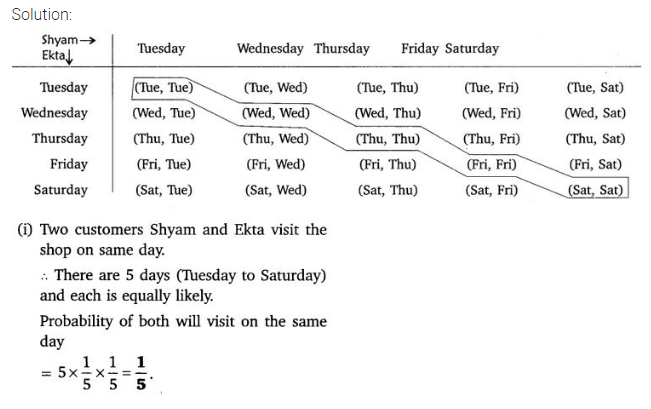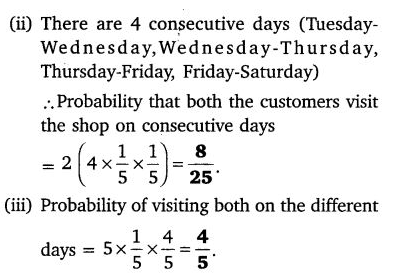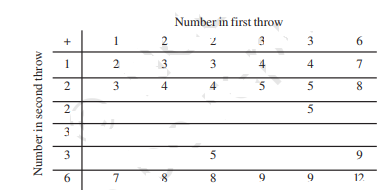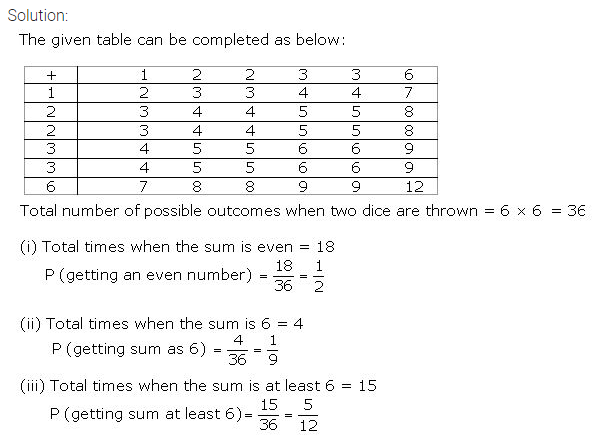• +91 9971497814
• info@interviewmaterial.com

# Probability Ex-15.2 Interview Questions Answers

### Related Subjects

Question 1 :

Two customers Shyamand Ekta are visiting a particular shop in the same week (Tuesday to Saturday). ?

Each is equally likely to visit the shop on any day as on another day. What is the probability that both will visit the shop on

(i) the same day?

(ii) consecutive days?

(iii) different daysQuestion 2 :

A die is numbered insuch a way that its faces show the numbers 1, 2, 2, 3, 3, 6. It is thrown twotimes and the total score in two throws is noted.

Answer 2 : Complete the following table which gives a few values of the total score on the two throws:What is theprobability that the total score is

(i) even?

(ii) 6?

(iii) at least 6?Question 3 :

A bag contains 5 redballs and some blue balls. If the probability of drawing a blue ball

is double that of ared ball, determine the number of blue balls in the bag.

It is given that the total number of red balls= 5

Let the total number of blue balls = x

So, the total no. of balls = x+5

P(E) = (Number of favourable outcomes/ Totalnumber of outcomes)

P (drawing a blue ball) = [x/(x+5)] ——–(i)

Similarly,

P (drawing a red ball) = [5/(x+5)] ——–(i)

From equation (i) and (ii)

x = 10

So, the total number of blue balls = 10

Question 4 :

A box contains 12balls out of which x are black. If one ball is drawn at random from the

box, what is theprobability that it will be a black ball?

If 6 more black balls are put in the box, the probability of drawing a black ball is now

double of what it was before. Find x

Solution:

Total number of black balls = x

Total number of balls = 12

P(E) = (Number of favourable outcomes/ Totalnumber of outcomes)

P (getting black balls) = x/12 ——————-(i)

Now, when 6 more black balls are added,

Total balls become = 18

Total number of black balls = x+6

Now, P (getting black balls) = (x+6)/18——————-(ii)

It’s given that, the probability of drawing a black ball now is double of what it wasbefore

(ii) = 2 × (i)

(x+6)/18 = 2 × (x/12)

x + 6 = 3x

2x = 6

x = 3

Question 5 :

A jar contains 24marbles, some are green and others are blue. If a marble is drawn at

random from the jar,the probability that it is green is ⅔. Find the number of blue balls

in the jar.

Total marbles = 24

Let the total green marbles = x

So, the total blue marbles = 24-x

P(getting green marble) = x/24

From the question, x/24 = ⅔

So, the total green marbles = 16

And, the total blue marbles = 24-x = 8

Todays Deals### Probability Ex-15.2 Contributorskrishan

Name:
Email:

# Latest News# 9000 interview questions in different categories